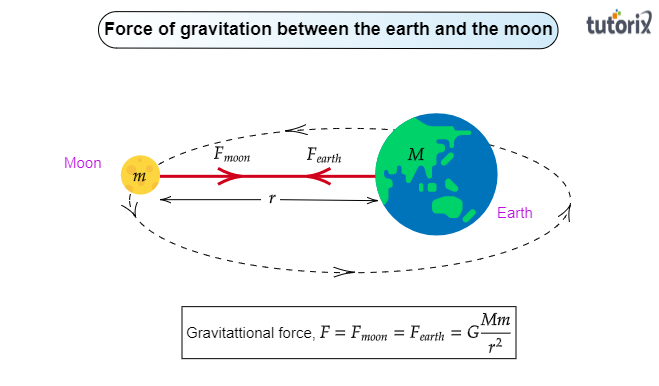# The earth and the moon are attracted to each other by gravitational force. Does the earth attract the moon with a force that is greater or smaller or the same as the force with which the moon attracts the earth? Why?

To do:

We have to check whether the Earth attracts the Moon with a force that is greater or smaller or with the same force that the Moon attracts the Earth.

Explanation:

The moon revolves around the earth due to the force of gravitation between them. To find out whether the force of attraction exerted by the earth on the moon is greater or less than or equal to the force of attraction exerted by the moon on the earth let us know, what the universal law of gravitation states about the force of gravitation between the two objects:

Universal law of gravitation:

Two objects placed at a certain distance attract each other with equal and opposite forces.

Force of gravitation between the earth and the sun:

The force of gravitation between the earth and moon is:

$F=F_{earth}=F_{moon}=G\frac{m_1m_2}{r^2}$Here, $F\rightarrow$ Force of gravitation between earth and moon

$F_{earth}\rightarrow$ Force exerted by the earth to attract the moon

$F_{moon}\rightarrow$ Force exerted by the moon to attract earth

$M\rightarrow$ Mass of the earth

$m\rightarrow$Mass of the moon

$r\rightarrow$ Distance between the earth and the moon

Based on the above discussion we can conclude that both the Moon and the Earth experience the same gravitational force from each other.